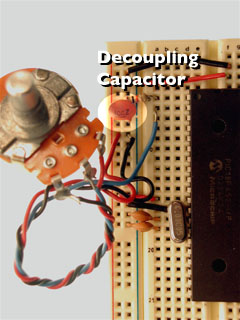While a digital input can tell us about discrete changes in the physical world, such as whether the cat is on the mat, or the cat is off the mat, there are times when this is not enough. Sometimes we want to know how fat the cat on the mat is. In order to know this, we’d need to be able to measure the force the cat exerts on the mat as a variable quantity. When we want to measure variably changing conditions like this, we need analog inputs. Many transducers are available to convert various changing conditions to changing electrical quantities. There are photocells that convert the amount of light falling on them to a varying resistance; flex sensors that change resistance as they are bent; Force-sensitive resistors (FSRs) that change resistance based on a changing force applied to the surface of the sensor; thermistors that change resistance in response to changing heat; and many more.

In order to read these changing resistances, we put them in a circuit and pass a current through them, so that we can see the changing voltage that results. There are a few variations on this circuit; the simplest uses a variable resistor. In the diagram on the left, we use a potentiometer (variable resistor with three connections). The center of the potentiometer (pot) is connected to the microcontroller. The other two sides are attached to power and ground. The resulting circuit is called a voltage divider. The ratio of the resistance on one side of the center of the pot to the resistance on the other side provides a varying voltage at the center of the pot.In the diagram on the right, we use a variable resistor from our voltage supply to the microcontroller pin, and a fixed resistor to ground. The variable resistor feeds a varying voltage to the microcontroller pin. The fixed resistor provides a path to ground. The current will follow the path of least resistance, from the 5V source through the variable resistor to the microcontroller pin. The higher the fixed resistor, the higher the bottom of the voltage range will be. Use a fixed resistor that’s in the same order of magnitude as the the range of the variable resistor. For example, if your variable resistor measures from 10 kilohms to 100 kilohms, use a 10 kilohm fixed resistor. If you build these circuits as shown above, and place your multimeter leads at the point that would go to the microcontroller and at ground, and measure for voltage, you should see a changing value.

Since a microcontroller’s inputs can read only two values (typically 0 volts or 5 volts), we need an extra component to read this changing, or analog voltage, and convert it to a digital form. An analog-to-digital converter (ADC) is a device that does this. It reads a changing input voltage and converts it to a binary value, which a microcontroller can then store in memory.

ADCs are a common tool, and many microcontrollers have ADCs built in to them. The BX-24 has eight ADCs built into it, attached to eight of its I/O pins. They are pins 13-20. The PIC 18F452 has eight ADCs as well, attached to pins RA0 through RA3, RA5, and RE0 through RE2. The Atmega8, which is the heart of the Arduino module, has 6. Many other microcontrollers have varying numbers of ADCs built in.

When the ADC circuitry attached to an ADC pin on these microcontrollers senses a varying voltage, it converts that voltage to a 10-bit number (0 to 1023). If you choose to read these pins using the ADC command instead of the getPin() command that we used to read them as a digital input, you will get that 10-bit number.

The resolution of an ADC changes from one ADC to another. However, both the BX-24 and most PICs with ADCs on board typically have 10-bit resolution. For more detail on the Analog special function registers, see the PIC analog In notes.

PicBasic Pro:

In order to read the ADCs on a PIC in PicBasic Pro, you need to configure a few of the special function registers on the PIC at the beginning of your program. The following defines will work on the 18F452, and most other ADC-equipped PICs:

```' Define ADCIN parameters:
DEFINE ADC_BITS 10 ' Set number of bits in result
DEFINE ADC_CLOCK 3 ' Set clock source (3=rc)
DEFINE ADC_SAMPLEUS 50    ' Set sampling time in uS

TRISA = %11111111 ' Set PORTA to all input
TRISE = %11111111 ' set port E to all input (40-pin PICs)
ADCON1 = %10000010 ' Set PORTA analog and right justify```

`ADCIN channel, analogVar`

channel is the ADC channel number: RA0 is channel 0, RA1 is channel 1, and so forth. Check the pin diagram for your chip to confirm the ADC pin numbers, they’ll be labelled AN0 through AN7 (or AN4 on 28-pin PICs).

analogVar is a word variable containing the result from the ADC.

BX-24

The command on the BX-24 is the getADC() command, and it looks like this:

`analogVar = getADC(pin)`

Pin is the BX-24 pin you are using;

analogVar is an integer variable containing the result from the ADC..

Wiring/Arduino

The command in Wiring is the analogRead() command, and it looks like this:

`analogVar = analogRead(pin)`